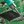# MOSFET TimerDIY Circuits wrote 05/02/2021 at 04:11 • 4 min read • Like

This article is about a MOSFET timer circuit. The LED and the light bulb turn ON after the button is pressed. They remain ON after the button is realised for about a minute, depending on the timer variable resistor setting.

You can see in the video that the circuit works well as a latch:

The circuit has a memory affect caused by charging and discharging for the capacitor.

Step 1: Design the Circuit

I drawn the circuit via old PSpice simulation software:

Calculate the time that the lights will be one after the button is released:

Time Constant = Ct*(Rt1 + Rt2)

= (100*10^-6)*(2,000,000+10,000)

= 201 seconds

This is a very approximate value because it depends on the specified value of the gate source voltage threshold that will turn ON or turn OFF the current flow. Lower gate source voltage ON threshold will keep the lights ON for longer. You can check the datasheets for minimum and maximum values.

Step 2: Simulations

Voltage simulations:

When the transistor gate source voltage (Vs - Vg) falls below 4 V  (6 V - 2 V), the current stops flowing from source to drain. You can see that this period if 100 seconds and not 200 seconds because the transistor turns OFF before the first time constant:

Vg (First Time Constant) = (Vs - Vs*exp(-1))

= (Vs - 0.36787944117*Vs)

= 6 V * (1 - 0.36787944117)

= 3.79272335297 V > 2 V

Step 3: Make the Circuit

I used a 47 uF capacitor instead of 100 uF capacitor. Do not use capacitors above 100 uF of the LED might take a long time to turn ON:

I also did not connect the timing resistors to keep the light ON longer. Because the input resistance of MOSFET is a few giga-ohms, the MOSFET will take a long time to discharge the capacitor and turn OFF the LEDs if Rt resistors are omitted.

Step 4: Attach 6 V Battery Holder

I attached a 6 V battery holder. The circuit might work with 4.5 V minimum because some MOSFETs do not need high gate voltages to turn ON.

Step 5: Testing

In the video I connected the blue wire to positive end of supply to discharge the capacitor and turn OFF the LED and light bulb.

Watch this video:

You must be careful not to short the positive and negative terminals. This might cause the battery to explode.

Conclusion

This is a very versatile circuit. You can see that without the Rt1 and Rt2 resistors the LED and the light bulb will remain ON for a very long time due to high gate impedance of the MOSFET.

## Discussions